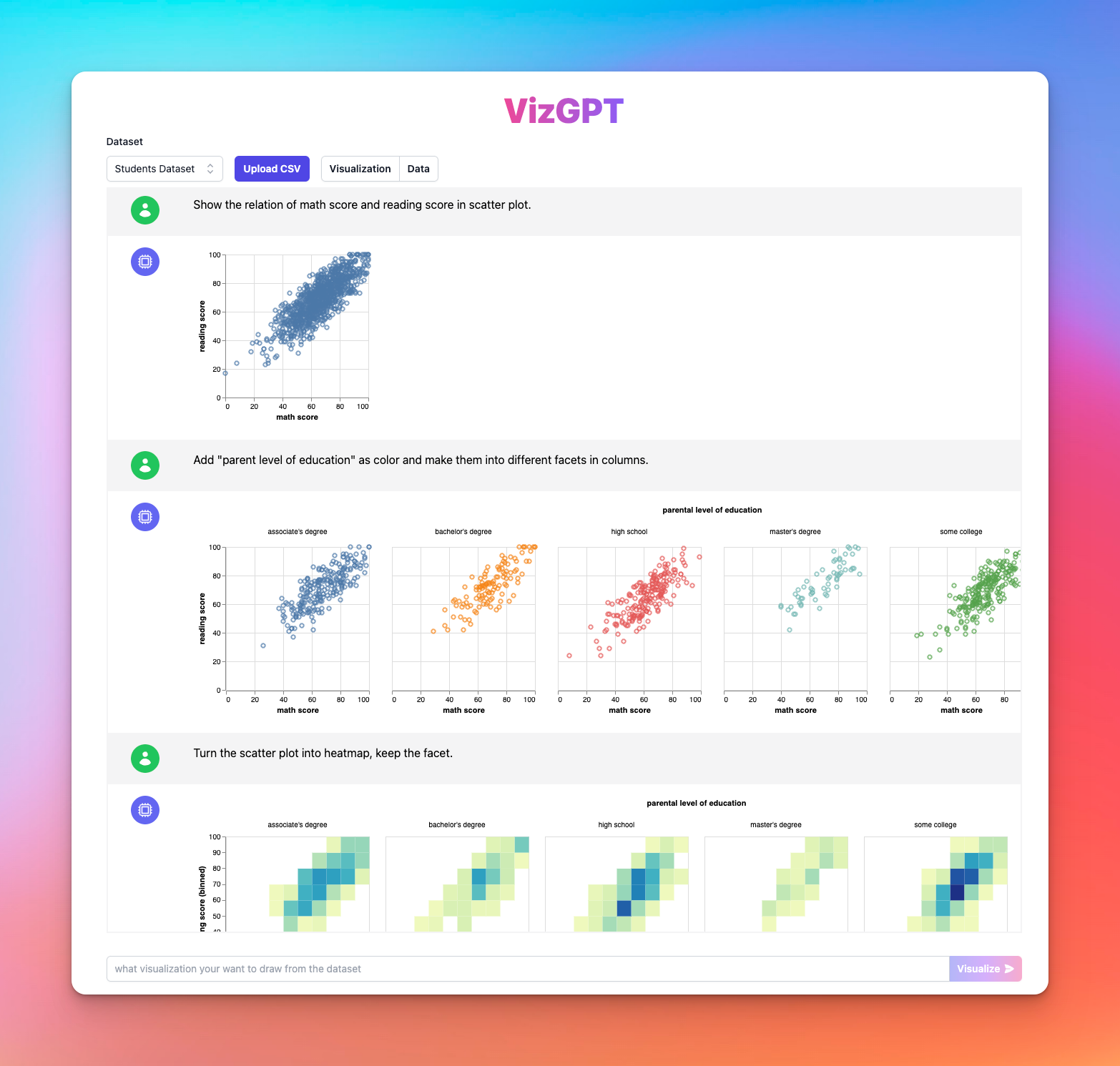# Mastering Pandas: DataFrames, CSVs, and More

In the world of data science and machine learning, Python has emerged as a front-runner due to its simplicity and powerful libraries. One such library is pandas. The pandas DataFrame, an essential tool for data manipulation and analysis, allows for efficient and intuitive handling of tabular data, becoming a mainstay in the Python programming language.

Before delving into pandas, let's take a moment to highlight a powerful tool called VizGPT (opens in a new tab), a chat interface that lets users create and edit data visualizations using natural language queries. This feature-rich tool can transform your data analysis, allowing you to explore data step-by-step through a chat-based interaction with your visualizations. Now, let's delve into the pandas DataFrame.

📚

## What is a pandas DataFrame?

A pandas DataFrame is a two-dimensional labeled data structure with columns of potentially different types. You can think of it like a spreadsheet or SQL table, or a dictionary of Series objects. It is generally the most commonly used pandas object and integrates seamlessly with many other data analysis tools and statistic software.

## Types of Data Structures in Pandas

Pandas primarily uses two types of data structures:

• Series: A one-dimensional labeled array capable of holding any data type. The axis labels are collectively referred to as the index.
• DataFrame: A two-dimensional labeled data structure with columns of potentially different types.

Both data structures are crucial when dealing with data analysis or scientific computing, but this tutorial will focus primarily on the pandas DataFrame.

## Creating a pandas DataFrame

Let's talk about the various methods of pandas DataFrame creation. You can create a DataFrame from:

• Dict of 1D ndarrays, lists, dicts, or Series
• 2-D numpy.ndarray
• A Series
• Another DataFrame

For instance, here's a quick pandas DataFrame example of creating a DataFrame from a dictionary:

``````import pandas as pd

data = {
'A': pd.Series([1, 2, 3]),
'B': pd.Series([1.0, 2.0, 3.0]),
'C': pd.Series(['a', 'b', 'c'])
}

df = pd.DataFrame(data)``````

You can also import data into a pandas DataFrame from CSV or TSV files using the `panda read csv` function. If you're dealing with a large dataset, consider:

• Python Modin, a scalable pandas DataFrame, to boost pandas DataFrame performance.
• PyGWalker (opens in a new tab), an Open Source Python Library that turns your Dataframes into a Tableau-like interface for creating Data Visualizations with No Code.

## Manipulating pandas DataFrame

Data manipulation is an integral part of data analysis. With pandas DataFrame manipulation, you can filter data, drop unnecessary data, or fill missing data using the `pandas fillna` function. To demonstrate, let's see how to drop a column in pandas:

``df.drop('A', axis=1)``

Additionally, pandas DataFrame provides the ability to perform mathematical operations on columns, such as calculating the standard deviation using `pandas stdev`.

## Visualizing Data with pandas

Pandas provides robust data visualization capabilities. You can generate various plots like bar plots, scatter plots, and more. For instance, you can create a scatter plot in

pandas using the `pandas plot scatter` function.

``df.plot.scatter(x ='A', y ='B')``

Also, you can use the `dataframe plot pandas` function for more advanced visualizations. However, for more advanced graphical representations, consider using a more dedicated tool, like VizGPT (opens in a new tab), to visualize data.(opens in a new tab)

## pandas DataFrame with Apache Spark and SQL Database

Pandas DataFrame also integrates well with Apache Spark, a distributed computing system, and SQL databases. With the `pandas to pyspark` function, you can convert a pandas DataFrame to a PySpark DataFrame, enabling you to distribute pandas workloads on Apache Spark.

Moreover, you can also interact with SQL databases. You can execute SQL queries directly on the pandas DataFrame, making it a versatile tool in database interactions and data extraction.

## Mastering pandas DataFrame

The true power of pandas lies in its versatility. From reading CSV files, manipulating data, performing statistical operations, plotting graphs, to integrating with distributed systems like Apache Spark and SQL databases, pandas DataFrame is an all-around user-friendly tool.

Pandas offers so much more than what's covered in this tutorial. Take time to explore this open-source library further, and consider using interactive coding platforms like ChatGPT Code Interpreter for practice. The journey to mastering pandas DataFrames will surely boost your data science skills and open doors to new possibilities.

## Pandas FAQ

1. What is a pandas DataFrame?

A pandas DataFrame is a two-dimensional labeled data structure with columns of potentially different types. It is like a spreadsheet or SQL table, or a dictionary of Series objects.

2. What types of data structures does pandas use?

Pandas primarily uses two types of data structures: Series and DataFrame.

3. How can I create a pandas DataFrame?

You can create a pandas DataFrame from a dictionary of 1D ndarrays, lists, dicts, or Series, a 2-D numpy.ndarray, a Series, or another DataFrame.

4. How do you delete indices, rows, or columns from a pandas DataFrame?

You can use the `drop` function of pandas DataFrame to delete indices, rows, or columns. For instance, `df.drop('A', axis=1)` will drop the column named 'A'.

5. Can you use pandas API on Apache Spark?

Yes, with the pandas to PySpark function, you can convert a pandas DataFrame to a PySpark DataFrame. This allows you to distribute pandas workloads on Apache Spark.

📚